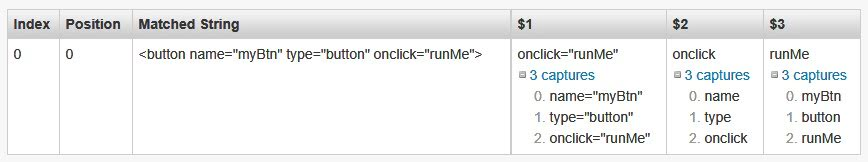﻿

# 正規表示式類別（Regex）獲取Match Group內的多重結果

## 利用正規表示式獲取群組（Groups）內的多重結果

``````<button name="myBtn" type="button" onclick="runMe">
``````

``````\<button(\s+(\S+)="(\S*)")*\>
``````

``````string cRegEx = @"\<button(\s+(\S+)=""(\S*)"")*\>";
string cInput = @"<button name=""myBtn"" type=""button"" onclick=""runMe"">";

string cResult = System.Text.RegularExpressions.Regex.Replace(cInput, cRegEx, oME =>
{
return \$"屬性名稱：{oME.Groups.Value} 屬性值：{oME.Groups.Value}";
});

WriteLine(cResult);
````````````string cRegEx = @"\<button(\s+(\S+)=""(\S*)"")*\>";
string cInput = @"<button name=""myBtn"" type=""button"" onclick=""runMe"">";

string cResult = System.Text.RegularExpressions.Regex.Replace(cInput, cRegEx, oME =>
{
string cTemp = string.Empty;
for (int i = 0; i < oME.Groups.Captures.Count; i++)
{
cTemp += \$"屬性名稱：{oME.Groups.Captures[i].Value} 屬性值：{oME.Groups.Captures[i].Value}\n";
}
return cTemp;
});

WriteLine(cResult);
``````

``````屬性名稱：name 屬性值：myBtn

``````